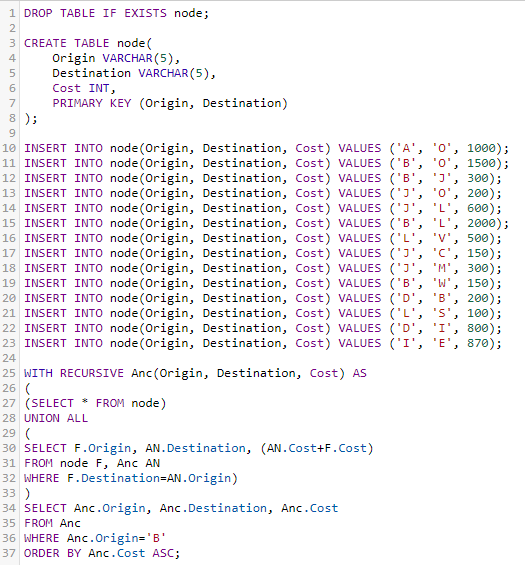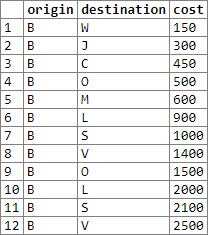# SQL Recursion: Common Table Expressions

Although I'm not really too interested in web languages, occassionally I come across interesting problems. The following problem is how to gather a list of connected nodes and add their edge weights. For instance:
if a -> b and b -> c, then a -> c
OR
a -> b and b -> c and a -> c then an edge exists directly from a to c

If each edge has a weight associated with it, we can then sum up the weight and say that for node A to travel to node C, it's the cost of a -> b + b -> c (provided there's no edge between a -> c). This type of problem can be solved recursively using common table expressions.In this example I look for all the nodes which "Node B" connects to; duplicate destinations mean different paths were found. So for instance B -> O appears twice because:
B -> O (1500)
AND
B -> J -> O (300 + 200 = 500)

Here are my results using Rextester, you can use my example by clicking the source code link above.